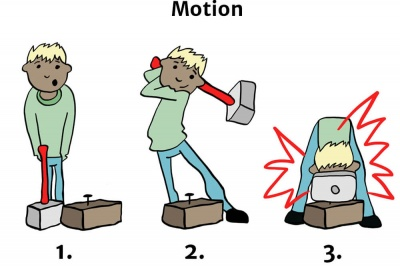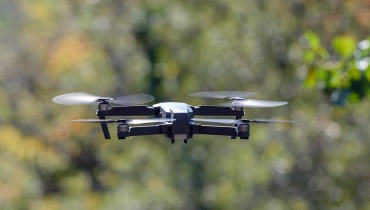# In a FlashBurning fuel moves a car, creating motion energy.

## Motion energy

Motion energy – also known as mechanical energy – is the energy stored in moving objects. As the object moves faster, more energy is stored.

Motion energy is the sum of potential and kinetic energy in an object that is used to do work.

# Burning Questions

## What is motion energy?

Motion – or mechanical – energy is the energy stored in moving objects.

## So motion energy must be a form of kinetic energy, right?

Well, not quite. Motion energy is actually the sum of kinetic and potential energy in an object that is used to do work.

## What do you mean by 'work'?

Work is when a force acts on an object and causes it to move, change shape or position, or do something physical.

When you push a door open, 'work' has been done on the door, causing it to move (open).The potential energy of a hammer + the kinetic energy of movement = motion energy.

## What's an example of motion energy in action?

Let's imagine you have an iron hammer and you want to use it to drive a nail into a piece of wood on the floor.

1. The iron hammer on its own has no kinetic energy, but it has some potential energy (because of its weight).
2. To do the work (i.e. drive the nail into the piece of wood), you first have to lift the hammer up – this increases the potential energy of the hammer because of its high position (see Gravitational Energy).
3. Next, you have to use force to move it down at great speed to hit the nail – so it now has kinetic energy.

The sum of the potential and kinetic energy that the hammer has acquired to drive in the nail is called motion energy – and that's what resulted in the work being done.

## What are some examples of motion energy?

Motion energy can be found in any moving object – here are some examples:WindA ball being thrownSomeone runningA fish swimming

We're sure you can think of heaps more - have a go!

# What Do You Mean?A Drone's electric motor is given electrical energy and it creates motion.

Motion energy is the sum of potential and kinetic energy in an object that is used to do work.

Work is when a force acts on an object and causes it to move, change shape, displace, or do something physical.

Potential energy is energy that is stored in an object or substance.

Kinetic energy is the energy of a moving object.

# Cool Facts

An electric motor makes mechanical energy from electrical energy, while a generator does the complete opposite.

# Speedy SummaryIn order for something to get moving, it must be pushed or pulled along.

Motion energy is the energy stored in moving objects. It is the sum of potential and kinetic energy in an object that is used to do work.

# Teacher's Toolkit

Take this to the classroom!